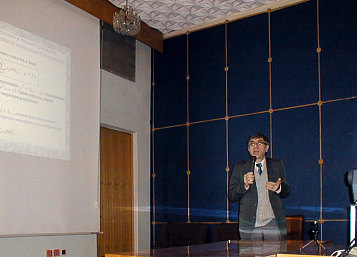B. Ts. Bakhshiyan
L- . MV- and E-optimal design of experiments: theory and simplex algorithms

 Main page Reports Discussion A mathematical analogy between a L-and MV-optimal experiments design and an ideal linear correction of system parameters is established, Optimality conditions are derived on the basis of this analogy and effective algorithms for solving these problems are designed. Mentioned algorithms are reduced to solutions of some generalized linear programming problems and its complexity not exceeds the usual linear programming problems complexity with m+1 ariables and m constraints - equalities, where m is the dimension of a estimated parameters vector in a linear regression model. The performance of these algorithms is demonstrated by solving a problem of a measurements optimal distribution for a polynomial regression, for which an analytical solution of the MV-optimal problem is found. The E-optimal problem is reduced to the generalized linear programming problem with M+1 by constraints, where M=m (m+1) /2. The difference derived solutions from the usual linear programming problem is that the optimality condition check is reduced to finding the eigenvalue of a MxM-matrix. The report contains outcomes of researches spent by Voiskovskii M. and Soloviov V. together with the author. Photos